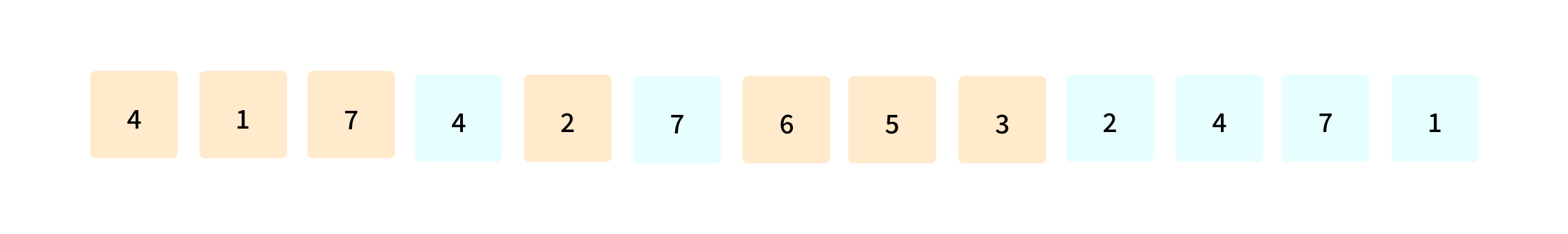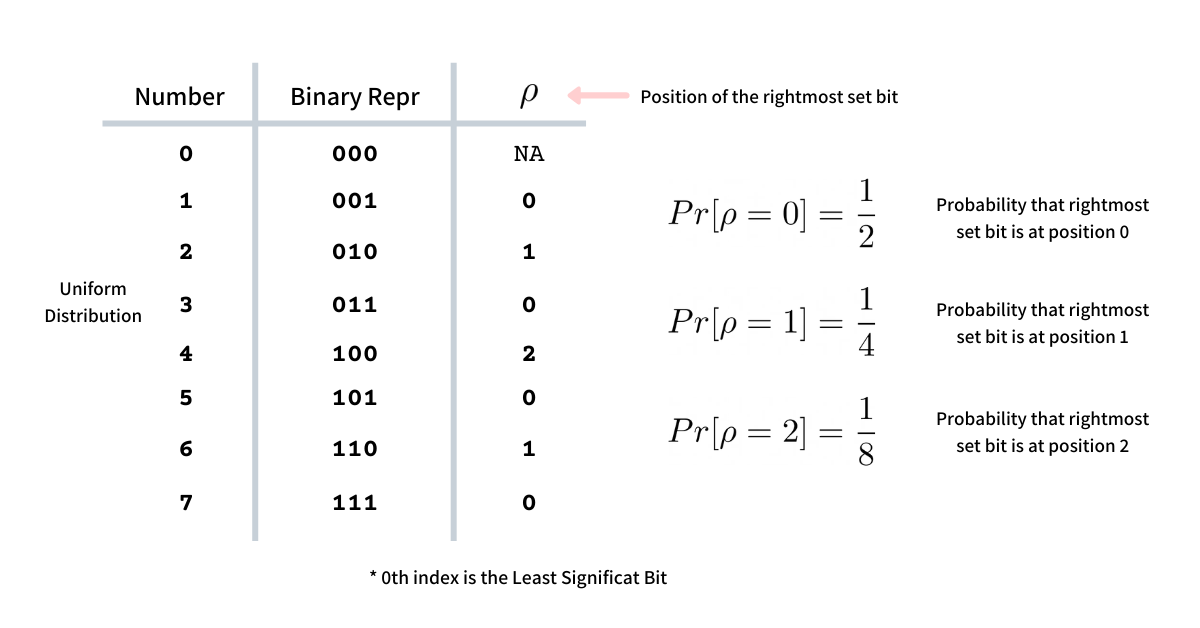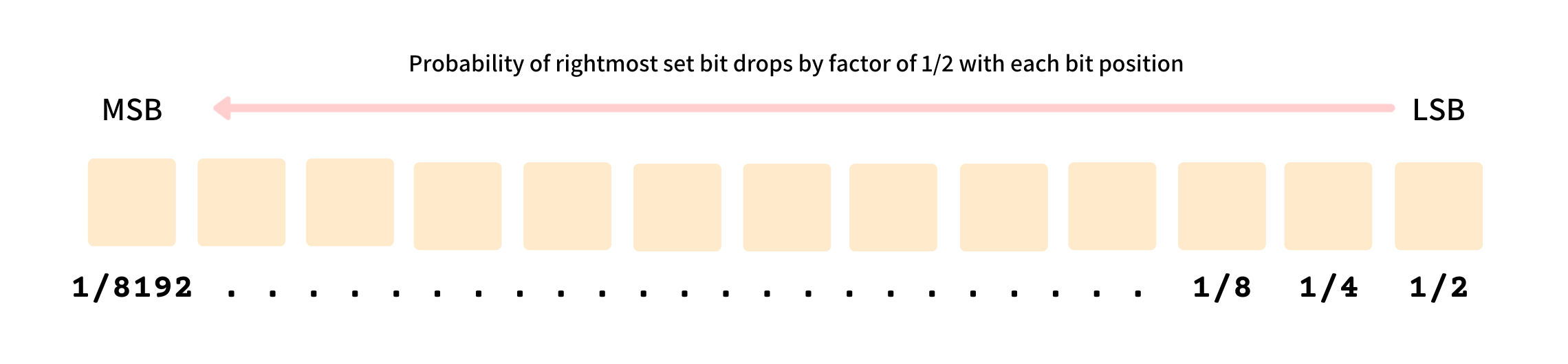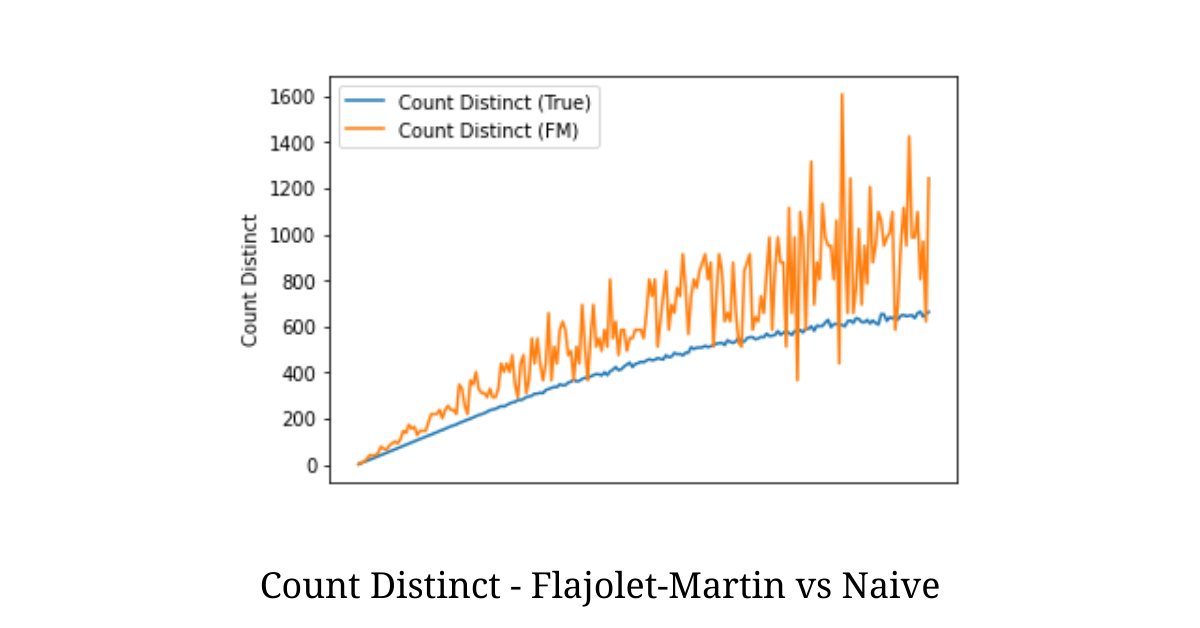Registrations for the November 2021 System Design Cohort are now open# Approximate Count-Distinct using Flajolet Martin Algorithm

Published on 6th Dec 2020

Measuring the number of distinct elements from a stream of values is one of the most common utilities that finds its application in the field of Database Query Optimizations, Network Topology, Internet Routing, Big Data Analytics, and Data Mining.

A deterministic count-distinct algorithm either demands a large auxiliary space or takes some extra time for its computation. But what if, instead of finding the cardinality deterministically and accurately we just approximate, can we do better? This was addressed in one of the first algorithms in approximating count-distinct introduced in the seminal paper titled Probabilistic Counting Algorithms for Data Base Applications by Philippe Flajolet and G. Nigel Martin in 1984.

In this essay, we dive deep into this algorithm and find how wittily it approximates the count-distinct by making a single pass on the stream of elements and using a fraction of auxiliary space.

# Deterministic count-distinct

The problem statement of determining count-distinct is very simple -

Given a stream of elements, output the total number of distinct elements as efficiently as possible.In the illustration above the stream has the following elements `4`, `1`, `7`, `4`, `2`, `7`, `6`, `5`, `3`, `2`, `4`, `7` and `1`. The stream has in all `7` unique elements and hence it is the count-distinct of this stream.

Deterministically computing count-distinct is an easy affair, we need a data structure to hold all the unique elements as we iterate the stream. Data structures like Set and Hash Table suit this use-case particularly well. A simple pythonic implementation of this approach is as programmed below

``````def cardinality(elements: int) -> int:
return len(set(elements))
``````

Above deterministic approach demands an auxiliary space of `O(n)` so as to accurately measure the cardinality. But when we are allowed to approximate the count we can do it with a fraction of auxiliary space using the Flajolet-Martin Algorithm.

# The Flajolet-Martin Algorithm

The Flajolet-Martin algorithm uses the position of the rightmost set and unset bit to approximate the count-distinct in a given stream. The two seemingly unrelated concepts are intertwined using probability. It uses extra storage of order `O(log m)` where `m` is the number of unique elements in the stream and provides a practical estimate of the cardinalities.

## The intuition

Given a good uniform distribution of numbers, the probability that the rightmost set bit is at position `0` is `1/2`, probability of rightmost set bit is at position `1` is `1/2 * 1/2 = 1/4`, at position `2` it is `1/8` and so on.In general, we can say, the probability of the rightmost set bit, in binary presentation, to be at the position `k` in a uniform distribution of numbers isThe probability of the rightmost set bit drops by a factor of `1/2` with every position from the Least Significant Bit to the Most Significant Bit.So if we keep on recording the position of the rightmost set bit, `ρ`, for every element in the stream (assuming uniform distribution) we should expect `ρ = 0` to be `0.5`, `ρ = 1` to be `0.25`, and so on. This probability should become `0` when bit position, `b` is `b > log m` while it should be non-zero when `b <= log m` where `m` is the number of distinct elements in the stream.

Hence, if we find the rightmost unset bit position `b` such that the probability is `0`, we can say that the number of unique elements will approximately be `2 ^ b`. This forms the core intuition behind the Flajolet Martin algorithm.

## Ensuring uniform distribution

The above intuition and approximation are based on the assumption that the distribution of the elements in the stream is uniform, which cannot always be true. The elements can be sparse and dense in patches. To ensure uniformity we hash the elements using a multiplicative hash functionwhere `a` and `b` are odd numbers and `c` is the capping limit of the hash range. This hash function hashes the elements uniformly into a hash range of size `c`.

## The procedure

The procedure of the Flajolet-Martin algorithm is as elegant as its intuition. We start with defining a closed hash range, big enough to hold the maximum number of unique values possible - something as big as `2 ^ 64`. Every element of the stream is passed through a hash function that permutes the elements in a uniform distribution.

For this hash value, we find the position of the rightmost set bit and mark the corresponding position in the bit vector as `1`, suggesting that we have seen the position. Once all the elements are processed, the bit vector will have `1`s at all the positions corresponding to the position of every rightmost set bit for all elements in the stream.

Now we find the position, `b`, of the rightmost `0` in this bit vector. This position `b` corresponds to the rightmost set bit that we have not seen while processing the elements. This corresponds to the probability `0` and hence as per the intuition will help in approximating the cardinality as `2 ^ b`.

``````# Size of the bit vector
L = 64

def hash_fn(x: int):
return (3 * x + 5) % (2 ** L)

def cardinality_fm(stream) -> int:
# we initialize the bit vector
vector = 0

# for every element in the stream
for x in skream:

# compute the hash value bounded by (2 ** L)
# this hash value will ensure uniform distribution
# of elements of the stream in range [0, 2 ** L)
y = hash_fn(x)

# find the rightmost set bit
k = get_rightmost_set_bit(y)

# set the corresponding bit in the bit vector
vector = set_bit(vector, k)

# find the rightmost unset bit in the bit vector that
# suggests that the probability being 0
b = rightmost_unset_bit(vector)

# return the approximate cardinality
return 2 ** b
``````

Although the above algorithm does a decent job of approximating count-distinct it has a huge error margin, which can be fixed by averaging the approximations with multiple hash functions. The original Flajolet-Martin algorithm also suggests that the final approximation needs a correction by dividing the approximation by the factor `ϕ = 0.77351`.

The algorithm was run on a stream size of `1048` with a varying number of distinct elements and we get the following plot.From the illustration above we see that the approximated count-distinct using the Flajolet-Martin algorithm is very close to the actual deterministic value.

A great feature of this algorithm is that the result of this approximation will be the same whether the elements appear a million times or just a few times, as we only consider the rightmost set bit across all elements and do not sample.

### Unique words in Thee Jungle Book

The algorithm was run on the text dump of The Jungle Book by Rudyard Kipling. The text was converted into a stream of tokens and it was found that the total number of unique tokens was `7150`. The approximation of the same using the Flajolet-Martin algorithm came out to be `7606` which in fact is pretty close to the actual number.

# References

If my work adds value, consider supporting me## 2000+ Subscribers

If you like what you read subscribe you can always subscribe to my newsletter and get the post delivered straight to your inbox. I write essays on various engineering topics and share it through my weekly newsletter 👇

# Other articles that you might likeSlowsort - A Pessimal Sorting Algorithm

Slowsort is a pessimal sorting algorithm based on the Multiply and Surrender paradigm. The algorithm...

26th Jul1D Procedural Terrain Generation

Terrains are at the heart of every Computer Game - be it Counter-Strike, Age of Empires, or even Min...

16th NovIsolation Forest Algorithm for Anomaly Detection

Anomaly detection is an age-old problem and in this article, we dive deep into an unsupervised algor...

28th JanMorris's Algorithm for Approximate Counting

Morris' Algorithm counts a large number of events using a very small space O(log log n). The algorit...

2nd Aug